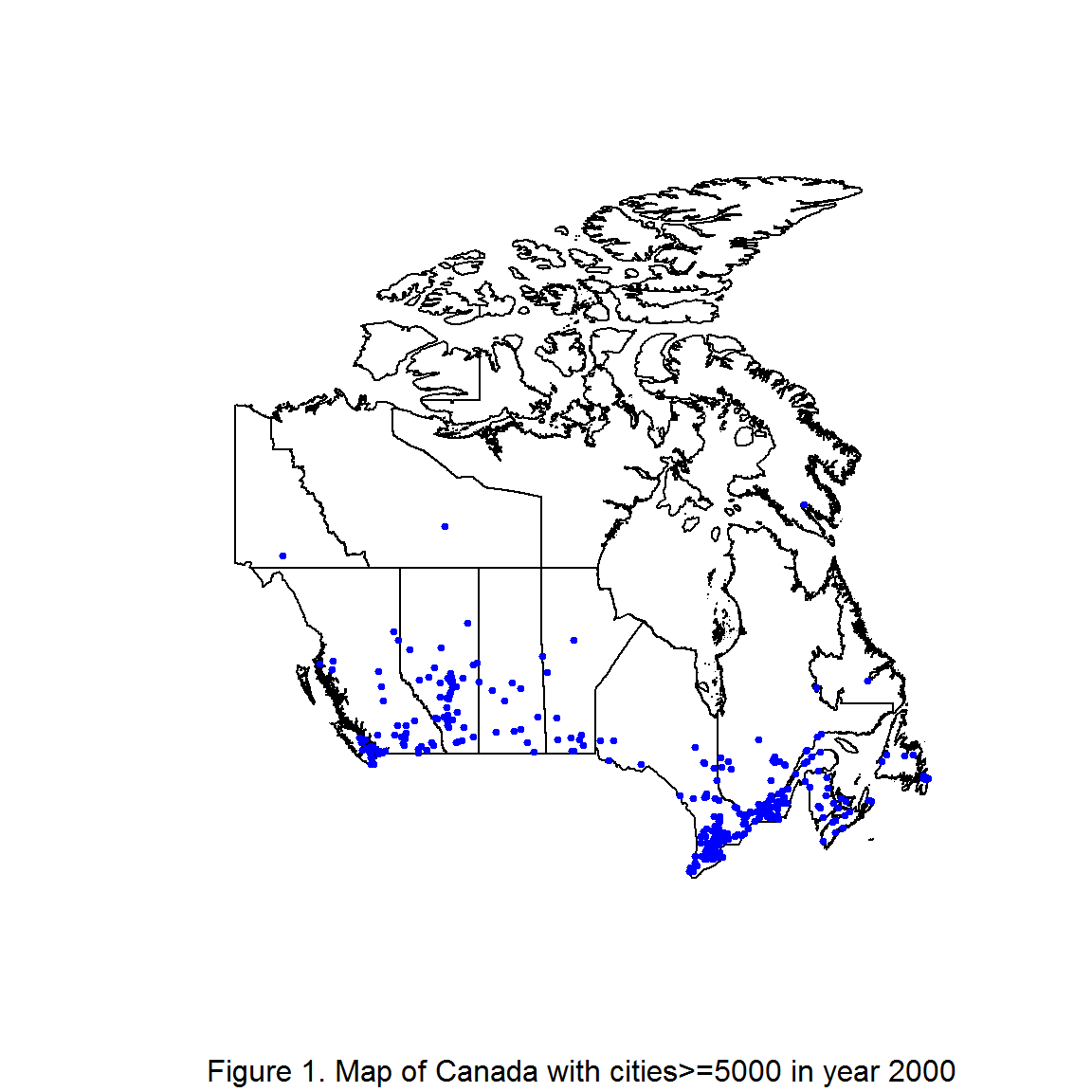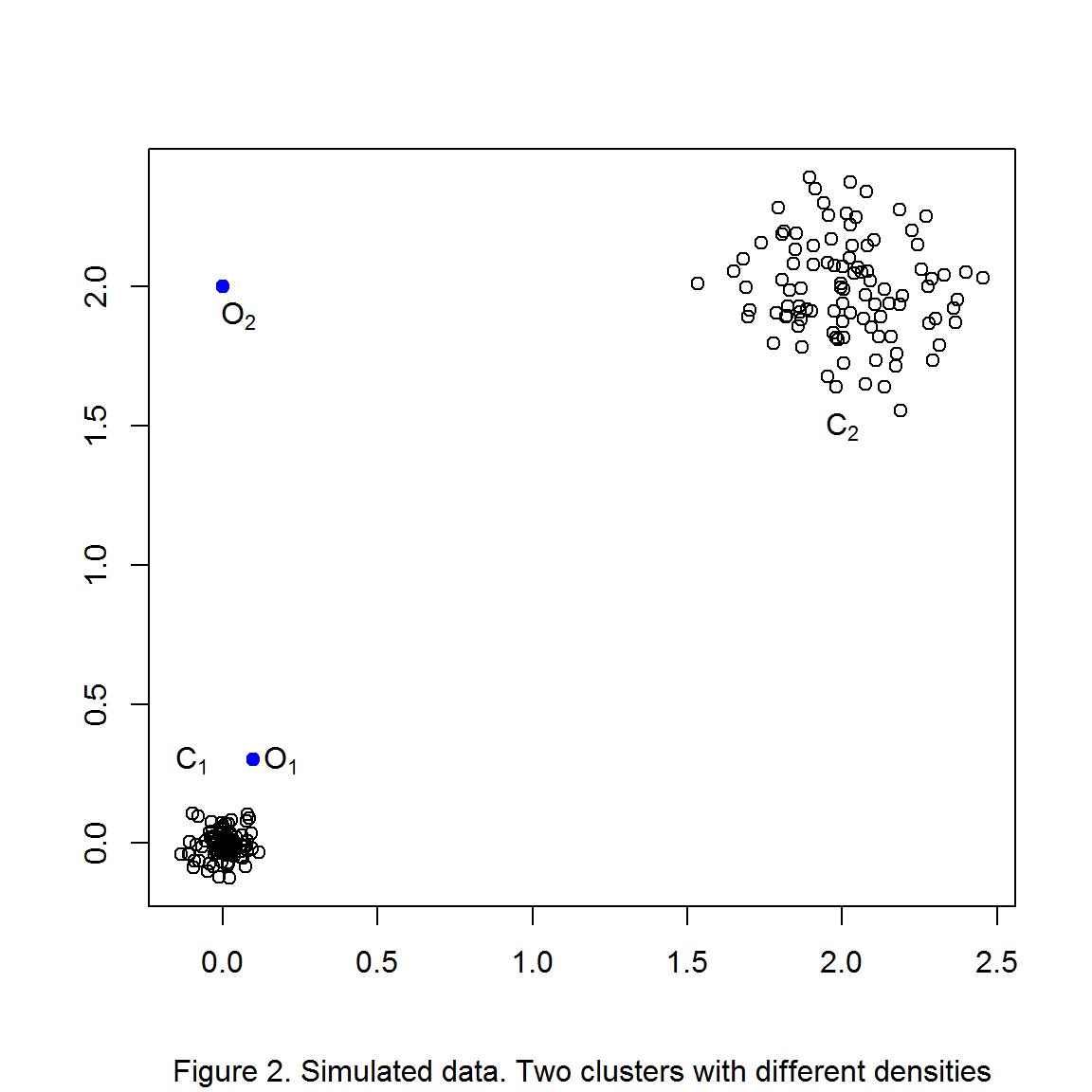## Friday, October 30, 2015

### LOF Outlying cities

LOF to find outlying cities (R code) (Part 1 of 2)

## Introduction

Canada has a concentrarion of relatively big cities in the south and sparsely located cities in the north. But, which cities show the most distinct behavior in terms of geographical location and population? In this post we will use LOF (Local Outlier Factor), a machine learning algorithm that uses neighborhoods, distances and densities to find outlying points. We will cover the main data analysis steps needed to find the most outlying cities: first data pre-processing , next an exploratory analysis, then the algorithm LOF, results section and finally the conclusion. As always remember that the objective of this blog is to explain the algorithm while using fun datasets(the “fun” factor is reader dependent!).

## Data Pre-processing

The dataset comes from http://www.tageo.com/index-e-ca-cities-CA.htm. I am using this data only because it was ready to use and to download, so i apologize if you find some innacuracies in the data, but again we are not here for the data but to understand the algorithm.
This dataset has 5 variables, but we will not use the first one (ranking of the cities), then the remaining 4 variables are:
• City (Factor). Name of the city.
• Population (Integer). Population of the city in year 2000.
• Latitude (Numeric). Geographical latitude.
• Longitud (Numeric). Geographical Longitude.
The dataset has information only about cities which population was >5000 in the year 2000.

## Exploratory analysis

First let’s explore the dataset with the str() function:
str(data)
## 'data.frame':    300 obs. of  4 variables:
##  $City : Factor w/ 300 levels "Abbotsford","Acton",..: 270 159 277 36 179 74 105 204 295 128 ... ##$ Population2000: int  4551800 3256300 1836500 938300 863000 812400 645100 643200 637900 403800 ...
##  $Latitude : num 43.6 45.5 49.3 51 45.4 ... ##$ Longitude     : num  -79.4 -73.6 -123.1 -114.1 -75.7 ...
Now, let’s plot a map of Canada and its main cities. First, we can obtain the data to outline Canada with the function getData() from the raster library, the data comes from http://gadm.org/. We can plot the map with the plot() function, this will take a minute or two depending on your computer. With the map plotted we can add the main cities of Canada using the points() function with the latitude and longitude as its x and y arguments. It is worth to mention that can_data (Map of Canada) is only used to have a visual aid that help us undersand the location of the cities, but obviously K-means will not use this information.
library(raster)
can_map<-getData('GADM', country="CAN", level=1) # provinces
plot(can_map,sub="Figure 1. Map of Canada with cities>=5000 in year 2000")
points(data$Longitud,data$Latitud,pch=19,col="blue",cex=.5)On observation of Figure 1, it is a clear a tendency to live in the south, maybe because we want to escape the coldest temperatures in the north (the winter is coming!), also the cities tend to form clusters with some some outlying cities in the north.

## The algorithm

Cluster analysis comprehend a class of algorithms that tries to classify similar observations into the same group. The main types of cluster algorithms are:
• Centroid based.
• Density based.
• Connectivity based.
In this analysis we are going to use LOF, a density based approach. The reason to use LOF is its ability to identify outliers depending on the local density and even small isolated groups of points. Outliers are then, to LOF, those points whose surrounding dentisy is different from the density around its neighbors. As example in Figure 2 there are two clusters with different densities($$C_{1}$$ and $$C_{2}$$) and 2 outliers($$O_{1}$$ and $$O_{2}$$), $$O_{2}$$ can be identified very easy by any clustering algorithm (like K-means), however things get a little more complicated with $$O_{1}$$. An algorithm like k-means could have difficulty detecting $$O_{1}$$, the reason for this is that the distances between neighbors in $$C_{2}$$ are very similar to the distances between $$O_{1}$$ and some points in $$C_{1}$$. Fortunately, we can use LOF to detect this type of outliers, LOF will take into account the difference in densities when computing the outlier scores.1. Breunig, M. M., H.-P. Kriegel, R. T. Ng, #246 and r. Sander (2000). “LOF: identifying density-based local outliers.” SIGMOD Rec. 29(2): 93-104.